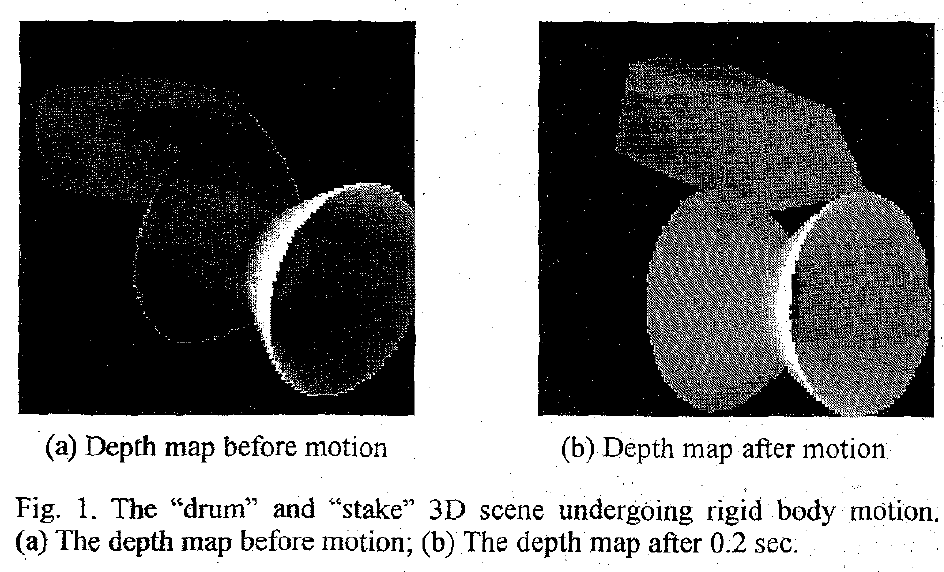# #13: Optic flow field segmentation and motion estimation using a robust genetic partitioning algorithm, , , and

IEEE Trans. Pattern Analysis and Machine Intelligence, Volume 17, pgs. 1177--1190, 1995

##### motion, tracking, segmentation, image analysis, machine learning

PlainText, Bibtex, PDF, URL, DOI, Google Scholar

#### Abstract

Optic flow motion analysis represents an important family of visual information processing techniques in computer vision. Segmenting an optic flow field into coherent motion groups and estimating each underlying motion is a very challenging task when the optic flow field is projected from a scene of several independently moving objects. The problem is further complicated if the optic flow data are noisy and partially incorrect. In this paper, the authors present a novel framework for determining such optic flow fields by combining the conventional robust estimation with a modified genetic algorithm. The baseline model used in the development is a linear optic flow motion algorithm due to its computational simplicity. The statistical properties of the generalized linear regression (GLR) model are thoroughly explored and the sensitivity of the motion estimates toward data noise is quantitatively established. Conventional robust estimators are then incorporated into the linear regression model to suppress a small percentage of gross data errors or outliers. However, segmenting an optic flow field consisting of a large portion of incorrect data or multiple motion groups requires a very high robustness that is unattainable by the conventional robust estimators. To solve this problem, the authors propose a genetic partitioning algorithm that elegantly combines the robust estimation with the genetic algorithm by a bridging genetic operator called self-adaptation